## date difference function in sql with example program

•  datediff sql function will helps to calculate the difference between two dates in week,year,months etc.
• datediff sql function accept the three arguments start_date, end_date and date_part.
•  date_part is a part of the date like year months and week that's compare between the start_date and end_date.
• start_date and end_date are two dates which is going to be compare.They contain the value in the form of  type DATE, DATETIME, DATETIMEOFFSET, DATETIME2, SMALLATETIME, or TIME.

#sql server datediff Return value of sql server datediff function

SQL DATEDIFF() function will return the integer value to indicate the  difference between the start_date and end_date and specified by the date_part.

sql server datediff function return the error if range of the integer return value is (-2,147,483,648 to +2,147,483,647).

# Example for  datediff SQL function  for SQL Server

•  let see the differences between two date value

Now use the DATEDIFF() function in SQL to compare two dates dates in various date parts:

```DECLARE
@start_dt DATETIME2= '2019-12-31 23:59:59.9999999',
@end_dt DATETIME2= '2020-01-01 00:00:00.0000000';

SELECT
DATEDIFF(year, @start_dt, @end_dt) diff_in_year,
DATEDIFF(quarter, @start_dt, @end_dt) diff_in_quarter,
DATEDIFF(month, @start_dt, @end_dt) diff_in_month,
DATEDIFF(dayofyear, @start_dt, @end_dt) diff_in_dayofyear,
DATEDIFF(day, @start_dt, @end_dt) diff_in_day,
DATEDIFF(week, @start_dt, @end_dt) diff_in_week,
DATEDIFF(hour, @start_dt, @end_dt) diff_in_hour,
DATEDIFF(minute, @start_dt, @end_dt) diff_in_minute,
DATEDIFF(second, @start_dt, @end_dt) diff_in_second,
DATEDIFF(millisecond, @start_dt, @end_dt) diff_in_millisecond;```

## OUTPUTDATEDIFF() function in SQL

# meshgrid matlab Function:-

Helps to generate X and Y matrices for three-dimensional plots.

## Syntax For meshgrid: -

```[X,Y] = meshgrid(x,y)
[X,Y] = meshgrid(x)
[X,Y,Z] = meshgrid(x,y,z)```

## Description for meshgrid matlab Function

• meshgrid function will help to trans transforms the given domain which is specified by the  x and y into arrays X and Y and these array can be used to check the two variables and three-dimensional mesh/surface plots.
•  Output rows of array X will be copies of x vector; same as the columns  output array Y will be copies of y vector
•  [X,Y] = meshgrid(x) is like [X,Y] = meshgrid(x,x).
•  [X,Y,Z] = meshgrid(x,y,z) helps to produces the three-dimensional arrays which is used to check the desired functions of three variables and three-dimensional volumetric plots.

# Important Note for meshgrid matlab Function

•  The meshgrid function in MATLAB is same as the ndgrid function but the point that should be remembered that is "in meshgrid function the order of  first two input and output arguments is switched"

[X,Y,Z] = meshgrid(x,y,z)

gives the same result as

[Y,X,Z] = ndgrid(y,x,z)

•   meshgrid function in MATLAB is surely suited for two- or three-dimensional Cartesian space.
•  ndgrid fucntion in MATLAB is suited for multidimensional problems that are not spatially based.

# Example for meshgrid matlab Function

```    [X,Y] = meshgrid(1:3,10:14)

X =

1     2     3
1     2     3
1     2     3
1     2     3
1     2     3

Y =

10    10    10
11    11    11
12    12    12
13    13    13
14    14    14

use meshgrid to create a surface plot of a function.

[X,Y] = meshgrid(-2:.2:2, -2:.2:2);
Z = X .* exp(-X.^2 - Y.^2);
surf(X,Y,Z)
```meshgrid to create a surface plot of a function.

## ord()Function in python with it's example

ord function in python is used to return an integer representing of Unicode character.

Syntax:-

`ord(ch)`

## Parameters For ord function in python

This function take just only one perameter and that known as ch (a Unicode character)

## Return value

The ord() function in python returns an integer representing the Unicode character.

## Example for ord()Function in python

```print(ord('5'))    # 53
print(ord('A'))    # 65
print(ord('\$'))    # 36```

## OUTPUTord()Function in python

## hasattr() function in python with example

Python hasattr() function in python return the true if the object gives the name of attribute and false if didn't return the name.

## Syntax for hasattr()

`hasattr(object, name)`

hasattr() in python called by the getattr() to check about the error

## Parameters For hasattr() function in python

There are two parameters which is used for this function the first one is object whose name is going to be checked.

And the name is second parameter which give the name for searched

## Return value

It gives boolean return value True and False

## Program Example For hasattr() in Python

```class Person:
age = 22
name = 'Adam stiffman'

person = Person()

print('Person has age?:', hasattr(person, 'age'))
print('Person has salary?:', hasattr(person, 'salary'))```

## Output

```Person has age?: True
Person has salary?: False```

## Use of Range Function In Python With Program

python range function in python return the sequence of numbers between the start integer value to the stop integer.

Syntax : -

```range(stop)
range(start, stop[, step])```

## Parameters For range() function in python:-

Range function takes the three arguments which is given below :-

•  first one is a start point which define the starting point of range function.
• second one is stop point integer which help to tell the end point for the sequence.
• Step is a third one and it use to define the increment for the sequence of number.

# Return value from range function in python: -

•  range() function return the immutable sequence of number.
• In this function sequence of number starts form 0 to Stop-1.
• if the step argument is then it raise an Value Error.

## How to use the range function in python with program

```# empty range
print(list(range(0)))

# using range(stop)
print(list(range(10)))

# using range(start, stop)
print(list(range(1, 10)))```

## fmincon Matlab Function With fmincon Example

fmincon function in MATLAB is used to find the minimum of any given nonlinear multivariable function.

MATLAB Syntax : -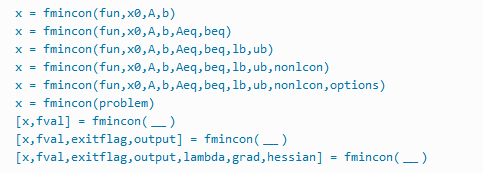MATLAB Syntax

## Description For fmincon MATLAB

Nonlinear programming solver.

Let see the a problem here and find the minimum value for this problem.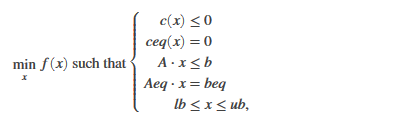fmincon MATLAB

Well b and beq are vectors, A and Aeq are matrices in this given equation on the other hand c(x) and ceq(x) are the functions in equation that return vectors.

well as you can see in the equation f(x) is a function which returns a scalar and remember all the three function f(x),ceq(x), and c(x) are non linear.

lb,x and ub can be passed as vectors or matrices in the fmincon MATLAB.

•  x = fmincon(fun,xθ,A,b) function is started with the xθ (x theta) and try to find the minimizer x for the given function and the expression for this  A*x ≤ b. xθ can be a scalar, vector, or matrix.
•  x = fmincon(fun,x0,A,b,Aeq,beq) minimize this function to linear equalities Aeq*x = beq and A*x ≤ b.If there is no inequalities exist, then set A = [] and b = [].
• x = fmincon(fun,x0,A,b,Aeq,beq,lb,ub) helps to define the lower and the upper case on the design variables in x, that's why the solution always exixt in between lb ≤ x ≤ ub.
x = fmincon(fun,x0,A,b,Aeq,beq,lb,ub,nonlcon) will helps to minimization to the nonlinear inequalities c(x) or the equalities ceq(x) defined in nonlcon.

x = fmincon(fun,x0,A,b,Aeq,beq,lb,ub,nonlcon,options) function helps to minimizes with the optimization options which is specified in options.

x = fmincon(problem) helps to finds the minimum for the given problem, and a structure described in problem.

[x,fval,exitflag,output] = fmincon(___) will helps to return a value exitflag that describes the exit condition of fmincon function MATLAB.

# Example for fmincon function MATLAB

Find the minimum value of Rosenbrock's function if there is a linear inequality constraint.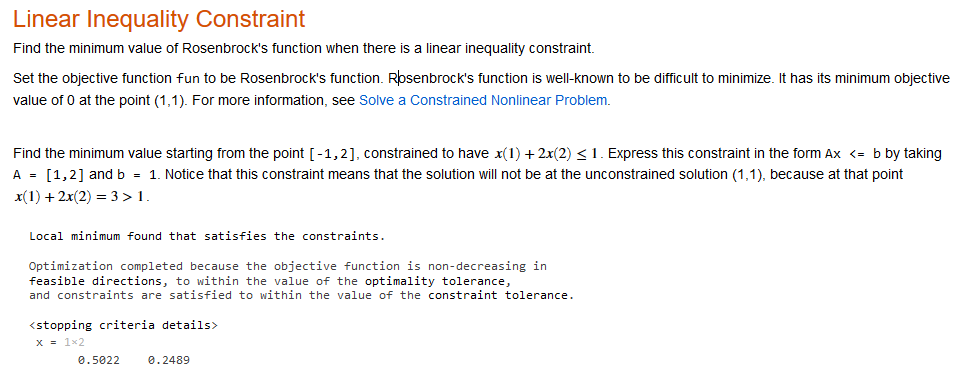Example for fmincon function MATLAB

## sprintf Function In MATLAB With Example Program

stg = sprintf(formatSpec,B1,...,Bn) use to formats the data in an array with the help of  formatting operators which is specified by formatSpec in MATLAB and returns the resulting text in a define variable(stg) then stg known as output and otherwise stg is an character.

MATLAB Program for Literal Text and Array Inputs

```formatSpec = 'The array is %dx%d.';
A1 = 2;
A2 = 3;
str = sprintf(formatSpec,A1,A2)```

compose function is used to return the number of pieces of formatted text as a string array and that can be used with the MATLAB sprintf() Function.

str = sprintf(literalText) In MATLAB use to translates escape-character sequences of the literalText which is define with sprintf function in MATLAB for example :-  \n and \t and sprint function return the all other  characters unaltered.

Now if the string contain any  formatting operator (example %f) then sprint function in MATLAB discards it and all characters after that operator.

## Input Arguments for sprintf function in MATLAB

• The Output is format with the help of  formatSpec and this also include the ordinary text and special characters.
• if  formatSpec is include the escape characters in string then  sprintf Function translates that escape characters (such as /n).

## Formatting Operator of sprintf

A formatting operator for sprintf starts with a percent sign, %, and ends with a conversion character and the conversion character is also required.

You can also specified some identifier like subtype operators, field width, precision, and  flags between % and  conversion character which is an end point.

## List Of Conversion Character for Sprintf function in MATLAB

There some of the Conversion CharacterConversion Character for Sprintf function

Some tips : -

The sprintf function in MATLAB is similar to fprintf function but the fprintf function is used for the Command Window.

## fprintf command And fprintf format in MATLAB

•  The fprintf command in MATLAB Programming use to displays formatted text which is centered on the icon and this fprint function can display formatSpec with a contents of var.
• `formatSpec`  in MATLAB can be a character vector with the single quotes, or a string scalar.

## Formatting for the fprint function in MATLAB : -

•  formatting is starts with the a percentage sign, % and it will end with the conversion character.
• Remember conversion character is required in formatting Operator.
• But you can use the  flags, field , identifier,width, and subtype,precision operators between % and conversion character.

## List for the Conversion Character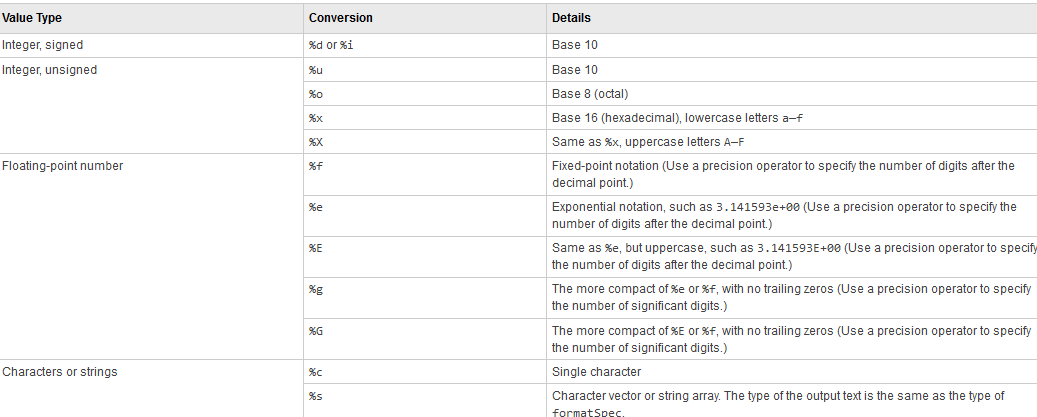Conversion Character in MATLAB

## Example of fprint function in MATLAB

```The command

fprintf('YES YES');

displays this text 'YES YES' on the icon.

The follow command

fprintf('YES YES = %d',16);

uses the decimal notation format (%d) to display the variable 16.```

## Using fprintf in MATLAB With Function

Let's see this example for define a function in MATLAB : -
```function []= fun2let(n)
if n > 90.00
fprintf('>>letter grade to %d is:A+\n',n)
elseif n<=89.49 && n>=80.00
fprintf('>>letter grade to %d is:B+\n',n)
% YOU COMPLETE YOUR FUNCTION WITH OTHER TESTS
elseif n<59.5
%    grade='Fail'
fprintf('>> letter grade to %d is:Fail\n',n)
end
end```

## frozenset() function in python With Example Code - Python Function

•  The frozenset() function in python is an inbuilt function is Python.
• That Function takes an iterable object as input and makes them immutable.
• it freezes the iterable objects and makes them unchangeable.
• frozen sets can be helpful in  Dictionary it can be used as key because it remain the same after creation.

## Parameter for the  frozenset() function in Python

it use a single parameter iterable which can be ( dictionary, tuple, etc.)

Return value : -

• The frozenset() function in python returns an immutable frozenset.
• it return the empty frozenset only if no parameters are passed to this function.

# Example code for frozenset function in python :-

```# tuple of vowels
vowels = ('a', 'e', 'i', 'o', 'u')

fset = frozenset(vowels)
print('The frozen set is:', fset)
print('The empty frozen set is:', frozenset())

# frozensets are immutable
fSet.add('v')```

## OUTPUTExample code for frozenset

## fft matlab Function And ftt code with Example

Fast Fourier transform of fft function in matlab.

## Syntax:-

```Y = fft(X)
Y = fft(X,n)
Y = fft(X,n,dim)```

## Discprition for fft matlab Function

If x is an vector then it will return the Fourier transform of that vector

Let x is an matrix then fft(X) assume the colum as a vactory and return Fourier transform of each column.

Now let assume X is an multidimensional array then fft(X) treats the values of first array dimension only if the size of that array is not equal to 1 as vector and gives the Fourier transform of each vector

# Cases with the fft() MATLAB Function

Y = fft(X,n) returns the n-point DFT.

## Case 1

X is an vector and the length of X is lower then n. X will be padded with trailing zeros to length n

## Case 2

X is an vector and the length of X is Higher then n. X will be truncated to length n

## Case 2

X is an matrix then each column of that matrix treated as in the vector case

## Case 2

multidimensional array X is treated as like fft(X) the "first array dimension only if the size of that array is not equal to 1" treated as in the vector case

Y = fft(X,n,dim) gives the Fourier transform along with the dimension dim.

## Example Code For ftt() function in MATLAB

Use the help of Fourier transforms to find the frequency components of a signal buried in noise.

```Fs = 1000;            % Sampling frequency
T = 1/Fs;             % Sampling period
L = 1500;             % Length of signal
t = (0:L-1)*T;        % Time vector
Form a signal containing a 50 Hz sinusoid of amplitude 0.7 and a 120 Hz sinusoid of amplitude 1.

S = 0.7*sin(2*pi*50*t) + sin(2*pi*120*t);

Corrupt the signal with zero-mean white noise with a variance of 4.

X = S + 2*randn(size(t));

Plot the noisy signal in the time domain. It is difficult to identify the frequency components by looking at the signal X(t).

plot(1000*t(1:50),X(1:50))
title('Signal Corrupted with Zero-Mean Random Noise')
xlabel('t (milliseconds)')
ylabel('X(t)')

Compute the Fourier transform of the signal.

Y = fft(X);

Compute the two-sided spectrum P2. Then compute the single-sided spectrum P1 based on P2 and the even-valued signal length L.

P2 = abs(Y/L);
P1 = P2(1:L/2+1);
P1(2:end-1) = 2*P1(2:end-1);

Define the frequency domain f and plot the single-sided amplitude spectrum P1. The amplitudes are not exactly at 0.7 and 1, as expected, because of the added noise. On average, longer signals produce better frequency approximations.

f = Fs*(0:(L/2))/L;
plot(f,P1)
title('Single-Sided Amplitude Spectrum of X(t)')
xlabel('f (Hz)')
ylabel('|P1(f)|')
Now, take the Fourier transform of the original, uncorrupted signal and retrieve the exact amplitudes, 0.7 and 1.0.

Y = fft(S);
P2 = abs(Y/L);
P1 = P2(1:L/2+1);
P1(2:end-1) = 2*P1(2:end-1);

plot(f,P1)
title('Single-Sided Amplitude Spectrum of S(t)')
xlabel('f (Hz)')
ylabel('|P1(f)|')
```

## OUTPUT For Example of fft () matlab:-fft () matlab outputfftshift matlabfourier transform matlab

## Learn about the Matlab linspace function With Example

Matlab linspace is used to Generate linearly spaced vector.

## Syntax for Matlabn Linspace: -

```y = linspace(x1,x2)
y = linspace(x1,x2,n)```

• y = linspace(x1,x2) give a row vector which is made with the 100 points with equal distance between x1 and x2
• y = linspace(x1,x2,n) return the n number of points and these point keep the distance of (x2-x1)/(n-1).
• This Matlab linspace function is very similar to the colon operator, “:” But linspace function in matlab direct control over the number of points and always include the endpoints.

# Example of Matlab linspace

```Create a vector of 100 evenly spaced points in the interval [-5,10].
y = linspace(-5,10);```

```Create a vector of 7 evenly spaced points in the interval [-5,7].
y1 = linspace(-5,7,7)```

## Important points : -

• x1 and x2 gives the interval for points which is generated by Matlab linspace.
• Data Types: single | double | datetime | duration
• Complex Number Support: Yes

## Example program for super function in python

super() function in python return the proxy object it's also a temporary object of the superclass and this object help us to access the method of base class.

Super function has two use :-

• This function Working with Multiple Inheritance
• Help us to avoid base class name explicitly

## Example of super function in python:-

if it use single inheritance then this function help us to refer base class by super()
```class Mammal(object):
def __init__(self, mammalName):
print(mammalName, 'is a warm-blooded animal.')

class Dog(Mammal):
def __init__(self):
print('Dog has four legs.')
super().__init__('Dog')

d1 = Dog()```

## OUTPUT

```Dog has four legs.
Dog is a warm-blooded animal.```

We can also change the name of base class Let see

```# changing base class to CanidaeFamily
class Dog(CanidaeFamily):
def __init__(self):
print('Dog has four legs.')

# no need to change this
super().__init__('Dog')```

Well super function in python is an call method for base class via delegation and that is called indirection and this happen at run then time in between this time we can also use the other base classes at different times.

## Example program for super() with Multiple Inheritance

```class Animal:
def __init__(self, Animal):
print(Animal, 'is an animal.');

class Mammal(Animal):
def __init__(self, mammalName):
print(mammalName, 'is a warm-blooded animal.')
super().__init__(mammalName)

class NonWingedMammal(Mammal):
def __init__(self, NonWingedMammal):
print(NonWingedMammal, "can't fly.")
super().__init__(NonWingedMammal)

class NonMarineMammal(Mammal):
def __init__(self, NonMarineMammal):
print(NonMarineMammal, "can't swim.")
super().__init__(NonMarineMammal)

class Dog(NonMarineMammal, NonWingedMammal):
def __init__(self):
print('Dog has 4 legs.');
super().__init__('Dog')

d = Dog()
print('')
bat = NonMarineMammal('Bat')```

## Method Resolution Order (MRO)For super() function in python

MRO is use to maintain the order at the persence of the multiple inheritance and ir can also view by using the __mro__ attribute.

```>>> Dog.__mro__
(<class 'Dog'>,
<class 'NonMarineMammal'>,
<class 'NonWingedMammal'>,
<class 'Mammal'>,
<class 'Animal'>,
<class 'object'>)
```

## Example program for setattr() function in Python

setattr() function in Python use to set the value of an attribute.setattr() function in Python

## Syntax : -

`setattr(object, name, value)`

If you want to read the object then use the getattr() function.

• Parameters For setattr():-

It takes three parameters and these are:-

• Object : - the object whose value is going to be set in python program.
• Name - set the name of attribute.
• value - Desire value given to the attribute

setattr() function in python doesn't return the value.

# Example program for setattr() function in Python

```class Person:
name = 'Adam'

p = Person()
print('Before modification:', p.name)

# setting name to 'John'
setattr(p, 'name', 'John')

print('After modification:', p.name)```

## Example for getattr() function in python

getattr() function in python is sued to get the value of an object attribute and it return the default value if no attribute of that object is found.Example for getattr() function in python

The reason of using getattr() function because it return the default value.Now let see the basic syntax : -

```getattr(object_name, attribute_name[, default_value])
```

## Example of getattr() function in python

this program will help you to teach about how to use the getattr() function in python

```class Student:
student_id=""
student_name=""

# initial constructor to set the values
def __init__(self):
self.student_id = "101"
self.student_name = "Adam Lam"

student = Student()
# get attribute values by using getattr() function
print('\ngetattr : name of the student is =', getattr(student, "student_name"))

# but you could access this like this
print('traditional: name of the student is =', student.student_name)
```

## Why use getattr() function

• This function helps to get the object attribute value with the help of Name of that attr. or you can also manually set the input attribute name by console.
• This function also give the freedom for set the default value according to your need.

## Example Program for delattr() function in python

Python delattr() function used to delete an attribute from an object.

## Syntax For delattr :-

`delattr(object, name)`

• ## second one is name it can be string which is removed from the object.

• delattr() function in python doesn't return any value.

# Python getattr Example Program in python:-

```class Coordinate:
x = 10
y = -5
z = 0

point1 = Coordinate()

print('x = ',point1.x)
print('y = ',point1.y)
print('z = ',point1.z)

delattr(Coordinate, 'z')

print('--After deleting z attribute--')
print('x = ',point1.x)
print('y = ',point1.y)

# Raises Error
print('z = ',point1.z)```

## Example Program for complie() function in python - Python Program

The complie() function in python is used to return python code object from a source like (string,AST object,byte string).

## Syntax For Complie(): -

`compile(source, filename, mode, flags=0, dont_inherit=False, optimize=-1)`

Well the return object from the compile function in python can be called by exec() and eval() method.

• ## A source that can be used in compile function that can be normal string, a byte string, or an AST object.

• A code that can be read from a file. If it doesn't read form a file then you give a name according to you.
• Either exec,eval or signal method can be used to call a function.
• Well eval accept only a single expression.
• exec take block of code with python statement like class function.
• it contain single interactive statement.

flags (optional) and dont_inherit : - if any problem stop to compile the source then it raise flag. default value : -0

optimize (optional) - optimization level with Default value -1 for compiler.Example Program for complie() function

# Example program for compile() function in python : -

```codeInString = 'a = 5\nb=6\nsum=a+b\nprint("sum =",sum)'
codeObejct = compile(codeInString, 'sumstring', 'exec')

exec(codeObejct)```

## OUTPUT

`sum = 11`

compile function in python convert the string into a python code object and then execute with exec() method

## Example Program For enumerate() function in python

enumerate() function in python adds counter to a iterable and returns it in the enumerate python program.

Syntax : -

`enumerate(iterable, start=0)`
• Two parameters are used the first one is an object which is accept the iteration.
• The second one is start parameters which is a starting point of counting but it's optional. If start is extinct the it's take 0 at start.
•   enumerate() function return the object after adding the counter to an iterable.

# Example Program for enumerate() in Python

```grocery = ['bread', 'Tea', 'jam']
enumerateGrocery = enumerate(grocery)

print(type(enumerateGrocery))

# converting the gienvalue into a list
print(list(enumerateGrocery))

# changing the default counter
enumerateGrocery = enumerate(grocery, 10)
print(list(enumerateGrocery))```

OUTPUTProgram for enumerate() in Python

## Example Program For Complex Function In Python - Python Program

•  Well Python not only handle the real number but also handle the complex numbers with the help of Complex function in python.
• This complex function is so useful to manipulate mathematics problem's.
• complex function in python return the real number from complex number or convert the string to a complex number.Example Program For Complex Function In Python

## Parameter : -

•     real - real part. If real is extinct then it defaults to 0.
•     imag - imaginary part. If imag is extinct then it defaults to 0.

## Example of Complex function in Python To Create a complex number:-

```z = complex(2, 3)
print(z)

z = complex(2)
print(z)

z = complex()
print(z)

z = complex('4-5j')
print(z)(2+3j)
(2+0j)
0j
(4-5j)```

## Example Program for vars() function in python - Python Example Program

vars() function in python return the dictionary attribute of an object.Example Program for vars() function in python

• vars() can just take one object that can be module, class, instance, or any object which  having the __dict__ attribute in python program.
• If the any object passed to vars() function doesn't  have the __dict__ attribute then it give an TypeError exception.
• If any argument doesn't pass to vars() function then it act like an locals() function.

# Example Program For vars function in python : -

```class Foo:
def __init__(self, a = 5, b = 10):
self.a = a
self.b = b

object = Foo()
print(vars(object))```

## OUTPUT

`{'a': 5, 'b': 10}`

## Example program for Any function in python - Python Example Program

Any function in python programming number of iterable is used and return the true if any element of any iterable is true or return the false.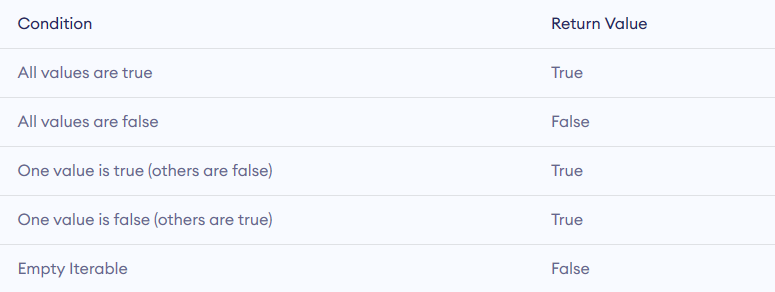Any function in python

## Syntax of any function in python

`any(iterable)`

Any function in python take iterable (string, dictionary etc.) as a Parameters.

Return : -

it's Return the boolean value.

•  True if any one element is true
•  Return the false if all the element are false.

# Example program for any() function in python : -

```l = [1, 3, 4, 0]
print(any(l))

l = [0, False]
print(any(l))

l = [0, False, 5]
print(any(l))

l = []
print(any(l))```

```True
False
True
False```

## Example program for any function on Python Strings

```s = "This is best"
print(any(s))

# 0 is False
# '0' is True since its a string character
s = '000'
print(any(s))

# False since empty iterable
s = ''
print(any(s))```